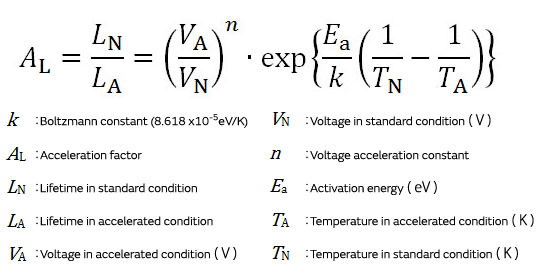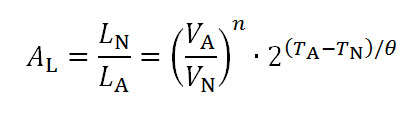# Ceramic Capacitors FAQ

Quality/Reliability

Are there any methods for estimating the lifetime of a capacitor?

Typical accelerated test for ceramic capacitor is an accelerated test using temperature and voltage factors. There have been many reports until now describing that acceleration equation for ceramic capacitor conforms to Eyring model. The duration period can be estimated by determining voltage acceleration constant(*1) and activation energy(*2).From this equation, by performing acceleration tests under more severe conditions (higher temperatures, higher voltages), the duration period can be estimated in environments where the product is actually used.

As simple estimating method, the following equation based on an empirical rule is also generally used :In the above expression, temperature difference θ between test temperature TA and standard (reference) temperature TN is referred to as temperature acceleration constant, wherein failure rate at TA becomes 1/2 (half) or 2 times (double) of failure late at TN.

Now, consider the comparison between the acceleration test of the multilayer ceramic capacitor and the estimated environment where the product is actually used. In this case, the above equation is used assuming the endurance test time in the acceleration test of the capacitor as LA, and the lifetime in standard conditions under the actual usage environment as LN.

Endurance test conditions Estimated usage conditions Voltage acceleration constant Temperature acceleration constant Lifetime in standard condition
TA＝85°C
VA＝20V
LA＝1000h
TN＝65°C
VN＝5V
n＝3
θ＝8 LN＝?h
As mentioned above, a 1,000-hour endurance test performed under an environment of 85ºC and 20V was estimated to be equivalent to 362039hrs (≒ 41 years!) under an environment of 65ºC and 5V. The voltage acceleration constant and temperature acceleration constant used in the equation differ by the type and structure of the ceramic material. Using the acceleration model allows for the verification of the duration period under actual usage environments over a long period, from the results of tests that take a relatively short time.

*1 Calculation of the voltage acceleration constant:
The target MLCC of evaluation is used for a sample, temperature applied during test is fixed, and life test by some applied voltage condition.
From the Weibull analysis result in each life test, the voltage acceleration constant “n” can be determined as the inclination of the approximate straight line of the relationship between logarithmic applied voltage and logarithmic mean time to failure (MTTF).

*2 Calculation of the activation energy:

Using the same sample as a voltage accelerated test, voltage applied during test is fixed, and life test by some temperature condition. From the Weibull analysis result in each life test, the activation energy “Ea” can be determined from the inclination of the approximate straight line of the relationship between reciprocal of absolute temperature and logarithmic mean time to failure (MTTF).

We appreciate your cooperation with the FAQ improvement questionnaire.